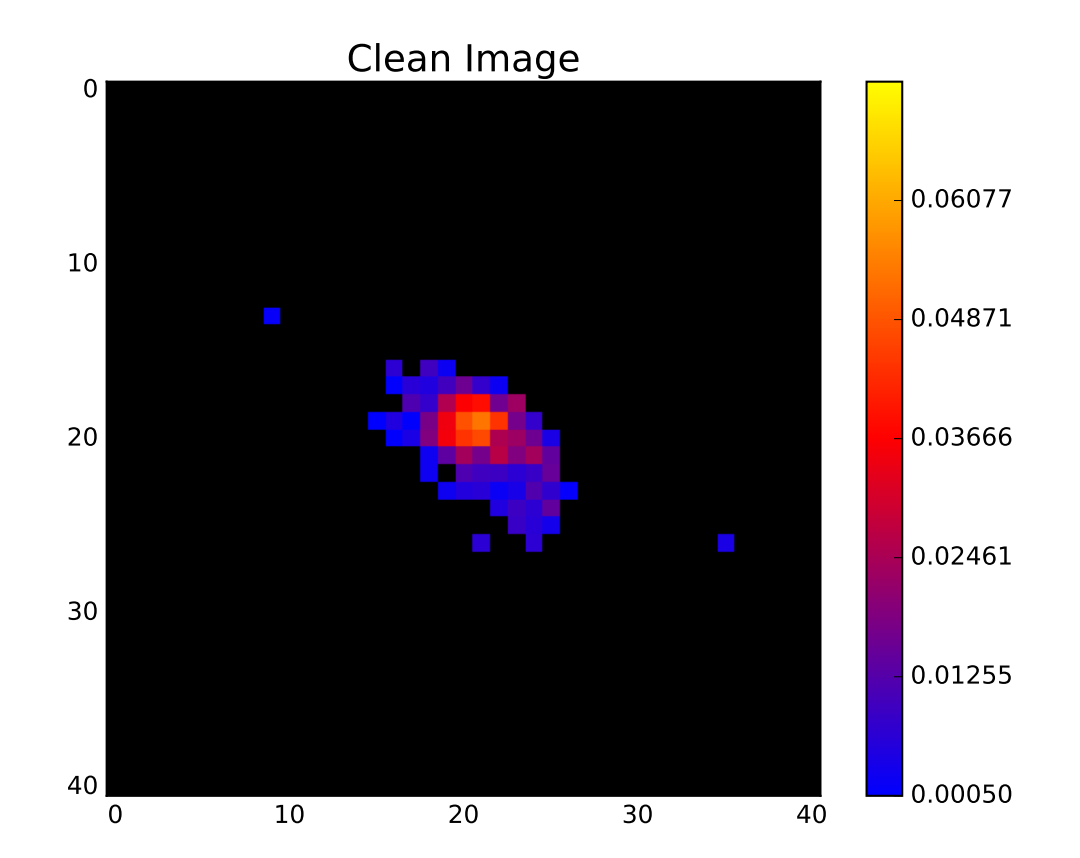# Space variant deconvolution of galaxy survey images## Abstract

Removing the aberrations introduced by the Point Spread Function (PSF) is a fundamental aspect of astronomical image processing. The presence of noise in observed images makes deconvolution a nontrivial task that necessitates the use of regularisation. This task is particularly difficult when the PSF varies spatially as is the case for the Euclid telescope. New surveys will provide images containing thousand of galaxies and the deconvolution regularisation problem can be considered from a completely new perspective. In fact, one can assume that galaxies belong to a low-rank dimensional space. This work introduces the use of the low-rank matrix approximation as a regularisation prior for galaxy image deconvolution and compares its performance with a standard sparse regularisation technique. This new approach leads to a natural way to handle a space variant PSF. Deconvolution is performed using a Python code that implements a primal-dual splitting algorithm. The data set considered is a sample of 10 000 space-based galaxy images convolved with a known spatially varying Euclid-like PSF and including various levels of Gaussian additive noise. Performance is assessed by examining the deconvolved galaxy image pixels and shapes. The results demonstrate that for small samples of galaxies sparsity performs better in terms of pixel and shape recovery, while for larger samples of galaxies it is possible to obtain more accurate estimates of the galaxy shapes using the low-rank approximation.

## Summary

The Point Spread Function or PSF of an imaging system (also referred to as the impulse response) describes how the system responds to a point (unextended) source. In astrophysics, stars or quasars are often used to measure the PSF of an instrument as in ideal conditions their light would occupy a single pixel on a CCD. Telescopes, however, diffract the incoming photons which limits the maximum resolution achievable. In reality, the images obtained from telescopes include aberrations from various sources such as:

• The atmosphere (for ground based instruments)
• Jitter (for space based instruments)
• Imperfections in the optical system
• Charge spread of the detectors

### Deconvolution

In order to recover the true image properties it is necessary to remove PSF effects from observations. If the PSF is known (which is certainly not trivial) one can attempt to deconvolve the PSF from the image. In the absence of noise this is simple. We can model the observed image $$\mathbf{y}$$ as follows

$$\mathbf{y}=\mathbf{Hx}$$

where $$\mathbf{x}$$ is the true image and $$\mathbf{H}$$ is an operator that represents the convolution with the PSF. Thus, to recover the true image, one would simply invert $$\mathbf{H}$$ as follows

$$\mathbf{x}=\mathbf{H}^{-1}\mathbf{y}$$

Unfortunately, the images we observe also contain noise (e.g. from the CCD readout) and this complicates the problem.

$$\mathbf{y}=\mathbf{Hx} + \mathbf{n}$$

This problem is ill-posed as even the tiniest amount of noise will have a large impact on the result of the operation. Therefore, to obtain a stable and unique solution, it is necessary to regularise the problem by adding additional prior knowledge of the true images.

### Sparsity

One way to regularise the problem is using sparsity. The concept of sparsity is quite simple. If we know that there is a representation of $$\mathbf{x}$$ that is sparse (i.e. most of the coefficients are zeros) then we can force our deconvolved observation $$\mathbf{\hat{x}}$$ to be sparse in the same domain. In practice we aim to minimise a problem of the following form

\begin{aligned} & \underset{\mathbf{x}}{\text{argmin}} & \frac{1}{2}\|\mathbf{y}-\mathbf{H}\mathbf{x}\|_2^2 + \lambda\|\Phi(\mathbf{x})\|_1 & & \text{s.t.} & & \mathbf{x} \ge 0 \end{aligned}

where $$\Phi$$ is a matrix that transforms $$\mathbf{x}$$ to the sparse domain and $$\lambda$$ is a regularisation control parameter.

### Low-Rank Approximation

Another way to regularise the problem is assume that all of the images one aims to deconvolve live on a underlying low-rank manifold. In other words, if we have a sample of galaxy images we wish to deconvolve then we can construct a matrix X X where each column is a vector of galaxy pixel coefficients. If many of these galaxies have similar properties then we know that X X will have a smaller rank than if images were all very different. We can use this knowledge to regularise the deconvolution problem in the following way

\begin{aligned} & \underset{\mathbf{X}}{\text{argmin}} & \frac{1}{2}\|\mathbf{Y}-\mathcal{H}(\mathbf{X})\|_2^2 + \lambda|\mathbf{X}\|_* & & \text{s.t.} & & \mathbf{X} \ge 0 \end{aligned}

### Results

In the paper I implement both of these regularisation techniques and compare how well they perform at deconvolving a sample of 10,000 Euclid-like galaxy images. The results show that, for the data used, sparsity does a better job at recovering the image pixels, while the low-rank approximation does a better job a recovering the galaxy shapes (provided enough galaxies are used).## Code

SF_DECONVOLVE is a Python code designed for PSF deconvolution using a low-rank approximation and sparsity. The code can handle a fixed PSF for the entire field or a stack of PSFs for each galaxy position.